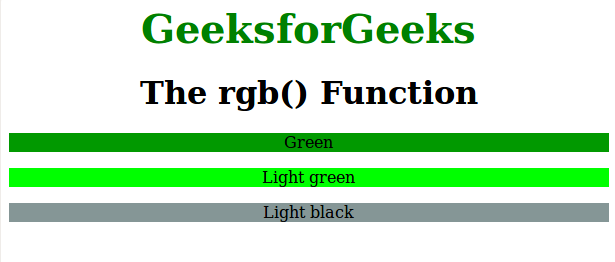Related Articles

# CSS | rgb() Function

• Last Updated : 04 Dec, 2018

The rgb() function is an inbuilt function in CSS which is used to define the colors using the Red Green Blue (RGB) model.

Syntax:

`rgb( red, green, blue )`

Parameters: This function accepts three parameters as mentioned above and described below:

• red: This parameter is used to define the intensity of red color. It is an integer value lies between 0 to 255, or as a percentage value between 0% to 100%.
• green: This parameter is used to define the intensity of green color. It is an integer value lies between 0 to 255, or as a percentage value between 0% to 100%.
• blue: This parameter is used to define the intensity of blue color. It is an integer value lies between 0 to 255, or as a percentage value between 0% to 100%.

Below programs illustrates the rgb() function in CSS:

Program:

 ```<``html``>``    ``<``head``>``        ``<``title``>rgb function``        ``<``style``> ``            ``.gfg1 {``                ``background-color:rgb(1, 153, 0);``                ``text-align:center;``            ``}``            ``.gfg2 {``                ``background-color:rgb(0, 255, 0);``                ``text-align:center``            ``}``            ``.gfg3 {``                ``background-color:rgb(133, 150, 150);``                ``text-align:center``            ``}``            ``.gfg {``                ``font-size:40px;``                ``font-weight:bold;``                ``color:green;``                ``text-align:center;``            ``}``            ``h1 {``                ``text-align:center;``            ``}``        ````    ````    ``<``body``>``        ``<``div` `class` `= ``"gfg"``>GeeksforGeeks``        ``<``h1``>The rgb() Function``        ``<``p` `class` `= ``"gfg1"``>Green``        ``<``p` `class` `= ``"gfg2"``>Light green``        ``<``p` `class` `= ``"gfg3"``>Light black``    ````                    `

Output:Supported Browsers: The browser supported by rgb() function are listed below:

• Chrome 1.0 and above
• Internet Explorer 4.0 and above
• Firefox 1.0 and above
• Safari 1.0 and above
• Opera 3.5 and above

Attention reader! Don’t stop learning now. Get hold of all the important HTML concepts with the Web Design for Beginners | HTML  course.

My Personal Notes arrow_drop_up